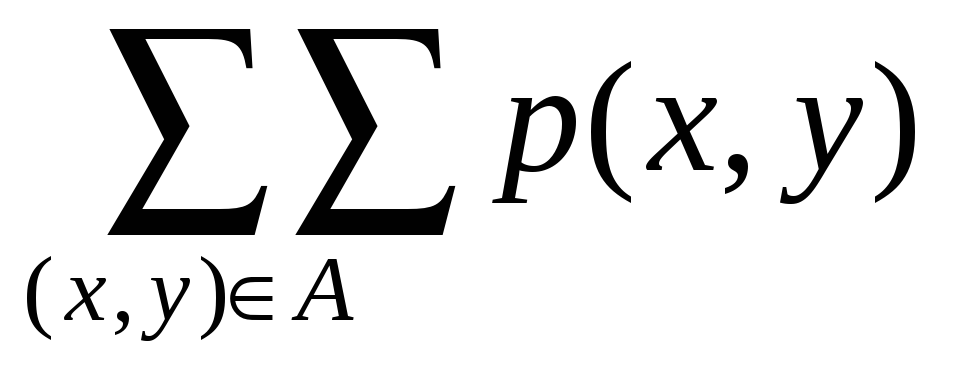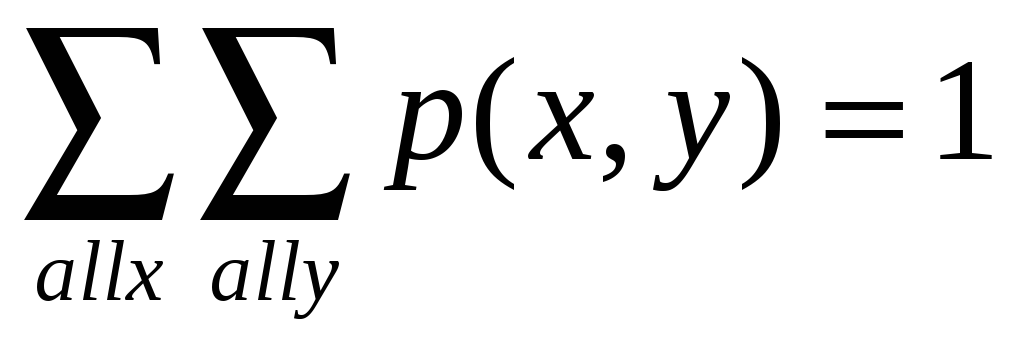# Chapter 5 Joint Probability Distributions and Random Samples

 Page 1/13 Date 19.05.2018 Size 1.16 Mb.

## Joint Probability Distributions and Random Samples

There are many experimental situations in which more than one random variables will be of interest to an investigator. Joint probability distribution will be considered for both discrete random variables and continuous random variables.

## Joint p.m.f. for two DISCRETE random variables

 Def. Let x and y be two discrete random variables defined on the sample space of an experiment. The joint probability mass functions p(x,y) is defined for each pair of numbers (x,y) by: p (x,y) = p (X = x, and Y = y) = fxy (x,y) Let A be any set consisting of pairs of (x,y) values. Then the probability P [(x, y) A] is obtained by summing the joint pmf over pairs in A: p [(x,y)  A] =Note A joint pmf must satisfy the following conditions.

p (x,y)  0(Row sum or column sum = 1)
Ex. 1 In an automobile plant, two tasks are performed by robots. The first entails welding two joints; the second, tightening three bolts. Let X denote the number of defective welds and Y the number of improperly tightened bolts produced per car. Past data indicates that the joint probability density for (X,Y) is as shown below.

 Y X 0 1 2 3 0 1 2 0.840 0.030 0.020 0.010 0.060 0.010 0.008 0.002 0.010 0.005 0.004 0.001

1. Find the probability that there will be no errors made by robots

2. Find the probability that there will be exactly one error

3. Find the probability that there will be no improperly tightened bolts